magnetic fields-the field of the compass (a permanent magnet) and the field around the conductor (a simple electromagnet). ">Custom SearchPERMANENT-MAGNET MOVING-COIL MOVEMENT The compass and conducting wire meter can be considered a fixed-conductor moving-magnet device since the compass is, in reality, a magnet that is allowed to move. The basic principle of this device is the interaction of magnetic fields-the field of the compass (a permanent magnet) and the field around the conductor (a simple electromagnet). A permanent-magnet moving-coil movement is based upon a fixed permanent magnet and a coil of wire which is able to move, as in figure 1-4. When the switch is closed, causing current through the coil, the coil will have a magnetic field which will react to the magnetic field of the permanent magnet. The bottom portion of the coil in figure 1-4 will be the north pole of this electromagnet. Since opposite poles attract, the coil will move to the position shown in figure 1-5. Figure 1-4. - A movable coil in a magnetic field (no current).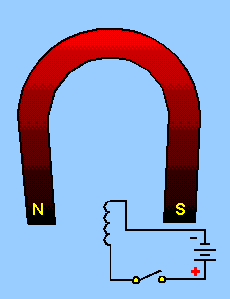Figure 1-5. - A movable coil in a magnetic field (current).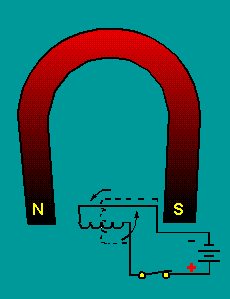The coil of wire is wound on an aluminum frame, or bobbin, and the bobbin is supported by jeweled bearings which allow it to move freely. This is shown in figure 1-6. Figure 1-6. - A basic coil arrangement.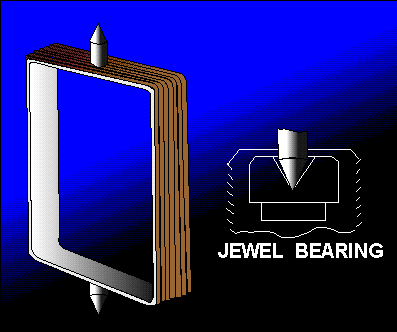To use this permanent-magnet moving-coil device as a meter, two problems must be solved. First, a way must be found to return the coil to its original position when there is no current through the coil. Second, a method is needed to indicate the amount of coil movement. The first problem is solved by the use of hairsprings attached to each end of the coil as shown in figure 1-7. These hairsprings can also be used to make the electrical connections to the coil. With the use of hairsprings, the coil will return to its initial position when there is no current. The springs will also tend to resist the movement of the coil when there is current through the coil. When the attraction between the magnetic fields (from the permanent magnet and the coil) is exactly equal to the force of the hairsprings, the coil will stop moving toward the magnet. Figure 1-7. - Coil and hairsprings.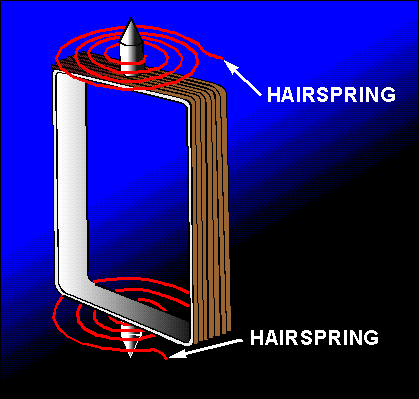As the current through the coil increases, the magnetic field generated around the coil increases. The stronger the magnetic field around the coil, the farther the coil will move. This is a good basis for a meter. But, how will you know how far the coil moves? If a pointer is attached to the coil and extended out to a scale, the pointer will move as the coil moves, and the scale can be marked to indicate the amount of current through the coil. This is shown in figure 1-8. Figure 1-8. - A complete coil.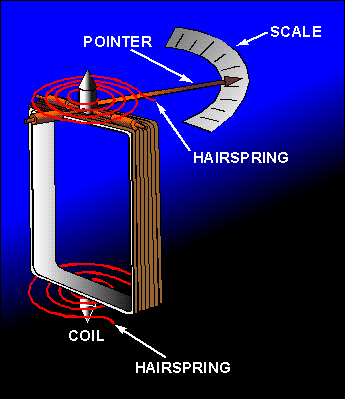Two other features are used to increase the accuracy and efficiency of this meter movement. First, an iron core is placed inside the coil to concentrate the magnetic fields. Second, curved pole pieces are attached to the magnet to ensure that the turning force on the coil increases steadily as the current increases. The meter movement as it appears when fully assembled is shown in figure 1-9. Figure 1-9. - Assembled meter movement.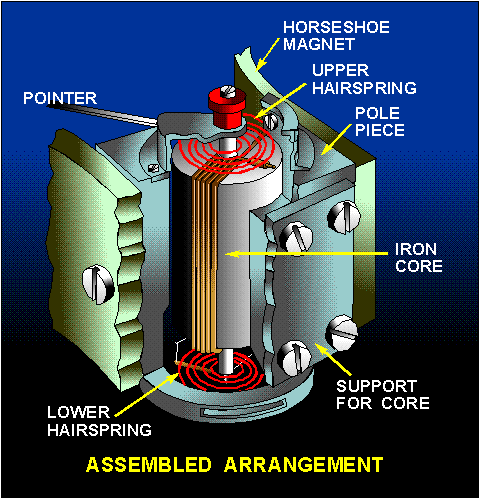This permanent-magnet moving-coil meter movement is the basic movement in most measuring instruments. It is commonly called the d'Arsonval movement because it was first employed by the Frenchman d'Arsonval in making electrical measurements. Figure 1-10 is a view of the d'Arsonval meter movement used in a meter. Figure 1-10. - A meter using d'Arsonval movement.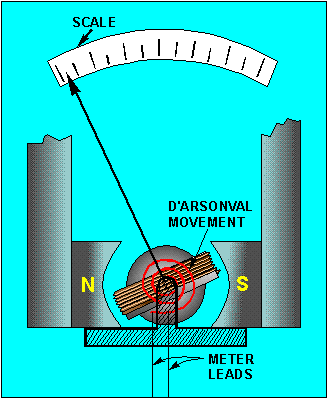Q.7 What type of meter movement is the d'Arsonval meter movement?Q.8 What is the effect of current flow through the coil in a d'Arsonval meter movement?Q.9 What are three functions of the hairsprings in a d'Arsonval meter movement?